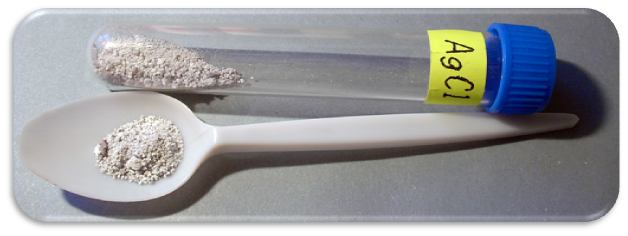## Molecular, Ionic, and Complete Ionic Equations

#### Learning Objective

• Recognize whether a chemical equation is written in molecular, ionic, or complete ionic form.

#### Key Points

• Chemical reactions that proceed through ionic forms can be written in a variety of ways.
• Molecular equations show species reacting as their molecular formula, with subscripts added to indicate their solid, liquid, gaseous, or aqueous nature.
• Ionic equations show species reacting as their ionic components. Subscripts are not needed to describe the state of the matter, because all ions are in aqueous solution. A net ionic equation is one in which spectator ions are removed.
• Spectator ions are present in solution but do not participate in the actual precipitation reaction.

#### Terms

• saltAn ionic compound that is composed of cations and anions. The constituent ions are held together by ionic bonds, not covalent bonds
• electrolytea substance that, when dissolved in solution, will enable the solution to conduct electricity
• spectator ionan ion that is present in solution but does not participate in a precipitation reaction

## Molecular Equation

There are various ways to write out precipitation reactions. In the molecular equation, electrolytes are written as salts followed by (aq) to indicate that the electrolytes are completely dissociated into their constituent ions; the (aq) designation indicates that the ions are in aqueous solution. For example, aqueous calcium chloride’s reaction with aqueous silver nitrate can be written as follows:

$CaCl_{2} (aq) + 2 AgNO_{3} (aq) \rightleftharpoons Ca(NO_{3})_{2} (aq) + 2 AgCl (s)$

On the right hand side of the equation, the precipitant (AgCl) is written in its full formula and designated as a solid, since this is the precipitate that is formed in the reaction. Note that the remaining salt, Ca(NO3)2, is still designed with (aq) to indicate that the ions are dissociated in solution.

## Complete Ionic and Net Ionic Equations

Because the reactants and one of the products are strong electrolytes, it is possible to write them out in terms of their constituent ions. The resulting equation is known as the complete ionic equation, and it looks as follows:

$Ca^{2+}(aq) + 2Cl^{-}(aq) + 2Ag{+}(aq) + 2NO_{3}^{-}(aq) \rightarrow Ca^{2+}(aq) + 2NO_{3}^{-}(aq) + 2 AgCl (s)$

In this equation, every ion is written out on both sides. The equation is balanced with the molar amount of each ion preceding it. This can be simplified to the net or complete ionic equation, which is shown below:

$2Cl^{-}(aq) + 2Ag^{+}(aq) \rightarrow 2AgCl (s)$

In this representation, the ions that are uninvolved in the precipitation reaction ($Ca^{2+}$ and $NO_{3}^{-}$) are simply excluded from the reaction, because they are essentially redundant on both the left and right side. In this particular instance, the equation can be further simplified to the reduced balanced form, with the cation coming before the anion as is most commonly practiced:

$Ag^{+}(aq) + Cl^{-}(aq) \rightarrow AgCl (s)$

The precipitated silver chloride appears as a white solid .Silver chlorideSilver chloride is a precipitant of silver and chloride ions reacting in solution.

The $Ca^{2+}$$NO_{3}^{-}$and the ions remain in solution and are not part of the reaction. They are termed spectator ions because they do not participate directly in the reaction; rather, they exist with the same oxidation state on both the reactant and product side of the chemical equation. They are only needed for charge balance of the original reagents.